Practice the questions of McGraw Hill Math Grade 4 Answer Key PDF Chapter 13 Test to secure good marks &amp; knowledge in the exams.

Write each missing number.

Question 1.
2 right angles = _____________
180

Explanation:
1 right angle = 90
2 right angles = 2 x 90 = 180
So, 2 right angles = 180.

Question 2.
3 right angles = _____________
270

Explanation:
1 right angle = 90
3 right angles = 3 x 90 = 270
So, 3 right angles = 270.

Use a protractor to measure each angle.

Question 3.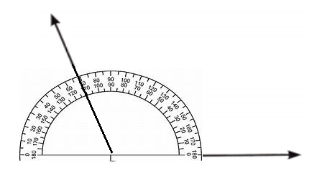Explanation:
The measure of the angle is 120-degrees.

Question 4.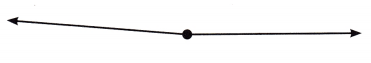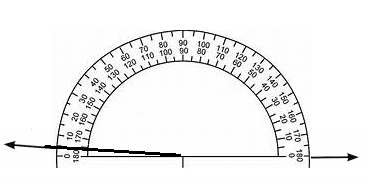Explanation:
The measure of the angle is 175-degrees.

Question 5.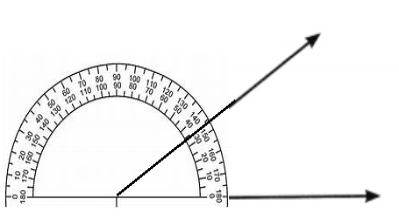Explanation:
The measure of the angle is 35-degrees.

Question 6.Explanation:
The measure of the angle is 80-degrees.

Solve. Write an equation for each.

Question 7.
∠ABC measures 34°.
∠CBD measures 113°.
What is the measure of ∠ABD?147°

Explanation:
∠ABC measures 34°
∠CBD measures 113°
34 + 113 = x
34 + 113 = 147
So, ∠ABD measures 147°

Question 8.
A wheel is turned 141° one way and then 49° the other way. At what degree measure does the wheel stop?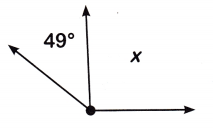92°

Explanation:
A wheel is turned 141° one way and then 49° the other way
141 – 49 = x
Subtract to find
141 – 49 = 92°
The wheel stops at 92° .

Draw an angle with each measure. Use a protractor.

Question 9.
50°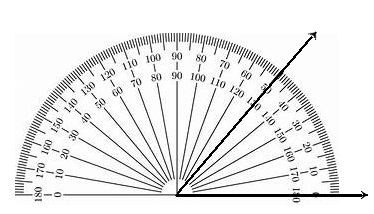Explanation:
The measure of the angle is 50-degrees.

Question 10.
145°Explanation:
The measure of the angle is 145-degrees.

Question 11.
97°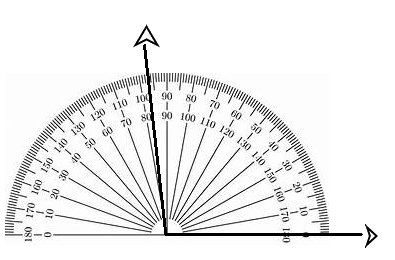Explanation:
The measure of the angle is 97-degrees.

Solve. Tell whether you used mental math, paper and pencil, or a calculator. Explain why.

Question 12.
Connor has a roll of tape. He uses 2$$\frac{1}{7}$$ ft to tape one present. He uses 4$$\frac{2}{7}$$ ft to tape another present. Then he uses 3$$\frac{2}{7}$$ feet to tape a third present. He has 14$$\frac{1}{7}$$ ft of tape left over. How many feet of tape did he begin with?
23$$\frac{6}{7}$$ ft

Explanation:
Connor has a roll of tape
He uses 2$$\frac{1}{7}$$ ft to tape one present
He uses 4$$\frac{2}{7}$$ ft to tape another present
Then he uses 3$$\frac{2}{7}$$ feet to tape a third present
He has 14$$\frac{1}{7}$$ ft of tape left over
2$$\frac{1}{7}$$ + 4$$\frac{2}{7}$$ + 3$$\frac{2}{7}$$ + 14$$\frac{1}{7}$$ = 23$$\frac{6}{7}$$
So, Connor begin with 23$$\frac{6}{7}$$ ft of tape.

Question 13.
A school group goes on a field trip to the Grand Canyon. 140 people ride 7 buses. If each bus holds the same number of people, how many people are on each bus?